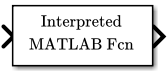# Interpreted MATLAB Function

MATLAB 函数或表达式应用于输入

• 库：

•## 说明

Interpreted MATLAB Function 模块用于将指定的 MATLAB® 函数或表达式应用于输入。函数的输出必须与模块的输出维度相匹配。

```sin atan2(u(1), u(2)) u(1)^u(2) ```

## 端口

### 输入

Interpreted MATLAB Function 模块接受一个 `double` 类型的实数或复数输入，并生成 `double` 类型的实数或复数输出，具体取决于 Output signal type 参数的设置。

### 输出

Interpreted MATLAB Function 模块接受一个 `double` 类型的实数或复数输入，并生成 `double` 类型的实数或复数输出，具体取决于 Output signal type 参数的设置。

## 模块特性

 数据类型 `double` 直接馈通 `是` 多维信号 `否` 可变大小信号 `否` 过零检测 `否`